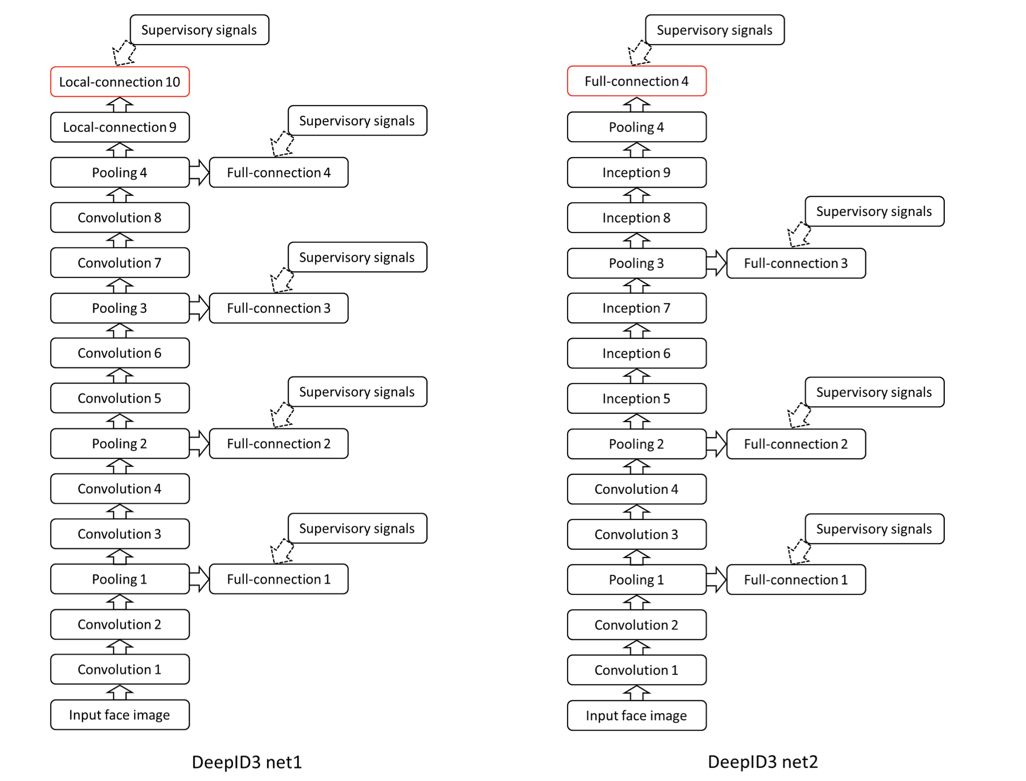DeepID系列

Posted by GwanSiu Blog on June 6, 2017

1.Introduction

Face recognition contains identification and verification. Identification is to classify an input image into a large number of identity classes, when verification is to classify a pair of images as belonging to the same identity or not.(i.e. binary classification). Identification is more challenging than verification. Because it’s more difficult to predict a training sample into one of many classes than to perform binary classification.

The basic philosophy of face recognition is to maximize inter-personal variations and minimize intra-personal variations. For example, fisher face approach is achieved this target. Another method is metric learning, which is to maps faces to some representations such that face of same identity is close to each other while those of different identities stay apart.

2.DeepID

In most face recognition algorithms, human face represents over-complete low-level feature based on shallow model. In DeepID, ConvNet takes a face patch as input and extracts high-level feature to represent human face. 200+ ConvNets(each ConvNets are corresponding to one patch) are utilized to train for identification, and then used trained ConvNet to extract face features and are feeded into join bayesian model for verification.
The arichitecture of DeepID ConvNets is followed: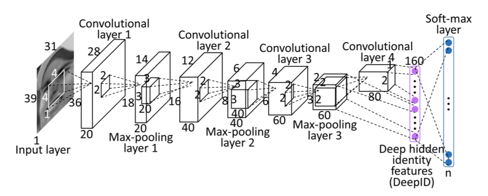The properties of DeepID:

1. The last hidden layer of DeepID is fully connected to both the third and fourth convolutional layers (after max- pooling) such that it sees multi-scale features. This is critical to feature learning because after successive down-sampling along the cascade, the fourth convolutional layer contains too few neurons and becomes the bottleneck for information propagation.

2. Feature numbers continues to reduce along the feature extraction hierachy until the last hidden layer(the DeepID layer).

3. Weights in higher convolutional layers of our ConvNets are locally shared to learn different mid- or high-level features in different regions.

How does the DeepID extract features for identity task?

1. Highly compact features(160 dimensions in this paper) are extracted in the top layer from the alternatively stack of convolutional layers. why to do so? Because it contrains DeepID to be significantly fewer than the classes of identities they predict, which is the key to learning highly compact and discriminative. It implicitly adds a strong regulatization to ConvNets, which helps to form shared hidden representations that can classify all the identities well.

2. weights in higher convolutional layer of our ConvNets are locally shared to learn differnent mid- or high-level feature in different regions.

3. The last hidden layer of DeepID is fully connected to both the third and fourth convolutional layers(after max-pooling) such that it sess multi-scale features.

DeepID 2

In DeepID2, supervisory signal is added to reduce the intra-personal variation. The basic idea of face recognition is to maximize inter-personal variation and minimize intra-personal variation at the same time. For DeepID, multi-classes classification(identificaiton, cross-entropy at the top layer) is to reduce the inter-personal variations. In DeepID2, verification signal is coorperated with the identificaiton signal to reduce the intra-personal variations.

The structure of neural network is the same as the DeepID, the main difference is that verification signal is added. Verification signal can be thought as regulatization, which regularizes the DeepID features to reduce the intra-personal variations.For identificaiton, the loss function is cross-entropy:

In this paper, author adopts two verification loss. One loss function is based on $L_{2}$ norm proposed by Hadesll. Another loss function is based on the cosine similarity.

$L_{2}$ norm loss function: $% $

where $f_{i}$ and $f_{j}$ are DeepID2 features vectors extracted from the two face images in comparision. $y_{ij}=1$ means that $f_{i}$ and $f_{j}$ are the same same person, while $y_{ij}=-1$ means that $f_{i}$ and $f_{j}$ are different person.$m$ is the distance margin. $\theta_{ve}$ is the verification loss parameters that can be learned in the training processing.

The loss function based on the cosine similarity:

where $d = \frac{f_{i}\bullet f_{j}}{\vert \vert f_{i}\vert \vert_{2} \vert \vert f_{j} \vert \vert_{2}}$ is the cosine similarity between DeepID2 feature vectors, $\theta_{ve} = {\omega,b}$ are learnable scaling and shifting arameters, $\sigma$ is the sigmoid function, and $y_{ij}$ is the binary target of whether the two compared face images belong to the same identity.

The goal is to learn the parameters $\theta_{c}$ in the feature extraction function Conv(·), while $\theta{id}$ and $\theta{ve}$ are only parameters introduced to propagate the identification and verification signals during training. All the parameters are undated by the gradient descent algorithms. In the testing stage, only $\theta_{c}$ are used to extract the features. $m$ is the loss function based on $L_{2}$ norm can’t be updated snice it callopses to zero. The algotithms is as followed: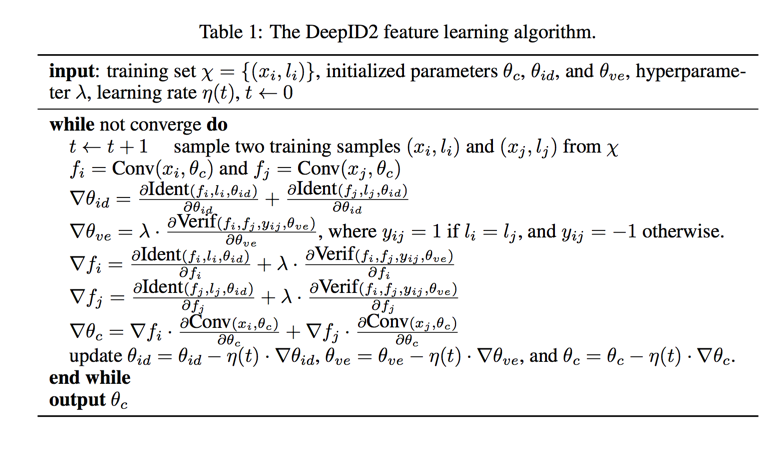What’s the mean of $\lambda$? Identification signal and Verification signal are changed when $\lambda$ is varied from $0$ to $\infty$. At $\lambda = 0$, the verification signal vanishes and only the identification signal takes effect. When $\lambda$ increases, the verification signal gradually dominates the training process. At the other extreme of $\lambda \rightarrow \infty$, only the verification signal remains.

DeepID2+

Compared with the DeepID 2, DeepID2+ added the supervisory signal in the early layers and increases the dimension of hidden repsresentation. In the DeepID 2+,Aauthor discover some nice property of neural network: sparsity, selecvtivity and robustness. The structure of network is as followed: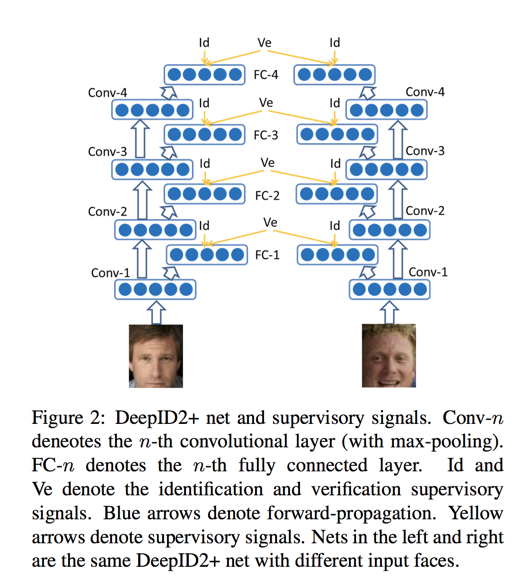What’s sparsity mean? It’s observed that neural activiation is sparse, and moderate sparsity can maximizes the discriminative power of deep neural network and increase the inter-personal distance. Therefore, DeepID2+ still acieve high performance after sparing the feature.

What’s the selectivity mean? It’s neurons in hidden layers are highly selective to the identity or identity-related attributes. The figure is shown this property.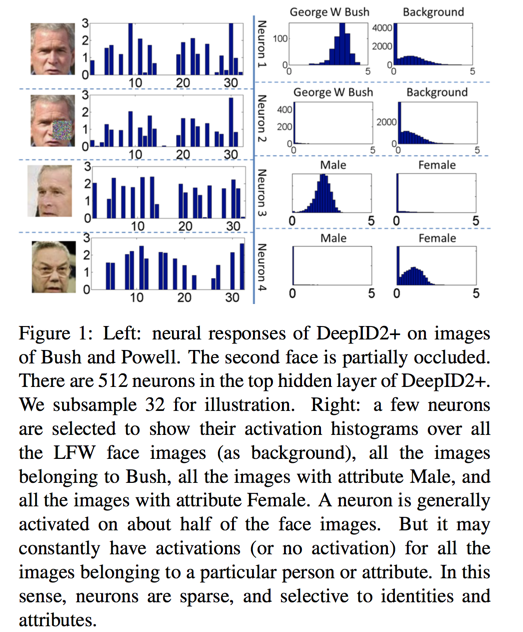DeepID 3

In DeepID3, author investigated how very deep structure of neural work influences the performace. In DeepID 3, it proposes two very deep neural networks one is to stacke convolutional layers and another one stacks inception layers at the top several layres instead of the convolutional layers.

The structure of DeepID 3 is as followed: